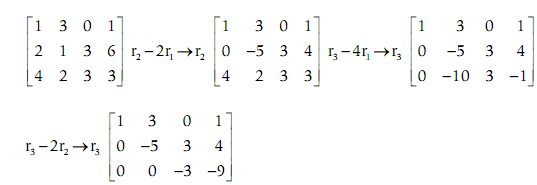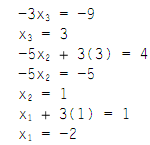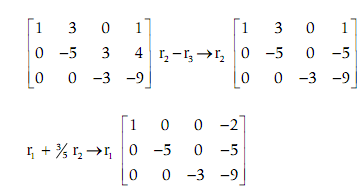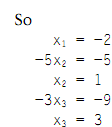## Forward substitution, MATLAB in Engineering

Assignment Help:

Forward substitution:

The Forward substitution (done methodically by first getting a 0 in the a21 place, and then a31, and lastly a32):For the Gauss technique, this is followed by the back-substitution:For the Gauss-Jordan technique, this is rather followed by the back elimination:#### Function issorted - set operations, Function issorted - set operations: ...

Function issorted - set operations: The function issorted will return 1 for logical true when the argument is sorted in ascending order (minimum to maximum), or 0 for false wh

#### Variable scope, Variable Scope: The scope of any of variable is the wo...

Variable Scope: The scope of any of variable is the workspace in which it is valid. The workspace generated in the Command Window is known as the base workspace. As we know

#### Illustration of image processing, Illustration of Image processing: Th...

Illustration of Image processing: This displays that there are 64 rows, or in another word, 64 colors, in this specific colormap. It also displays that the first five colors a

#### Anonymous functions, Anonymous Functions: The anonymous function is a ...

Anonymous Functions: The anonymous function is a very easy, one-line function. The benefit of an anonymous function is that it does not have to be stored in an M-file. This ca

#### Illustration of symbolic variable, Illustration of symbolic variable: ...

Illustration of symbolic variable: When, on the other hand, z is a symbolic variable to start with, quotes are not required around the expression, and the words are automatica

#### Function cellplot - cell array, Function cellplot - Cell array: The fu...

Function cellplot - Cell array: The function cellplot place a graphical display of the cell array in a figure Window; though, it is a high-level view and fundamentally just di

#### Plotting streamline in matlab, I have a vector of X, one for Y , one for x-...

I have a vector of X, one for Y , one for x-direction velocity U and one for y-direction velocity V. they are at same size. How can I plot streamline of that flow? I follow all exa

#### Logical scalar values - operators, Logical scalar values: The MATLAB a...

Logical scalar values: The MATLAB also has or and and operators which work element wise for the matrices: These operators will compare any of the two vectors or matric

#### Matrix multiplication, Matrix Multiplication: The Matrix multiplicatio...

Matrix Multiplication: The Matrix multiplication does not mean multiplying term by term; and it is not an array operation. The Matrix multiplication has a very particular mean

#### Illustration of gauss elimination, Illustration of Gauss elimination: ...

Illustration of Gauss elimination: For illustration, for a 2 × 2 system, an augmented matrix be: Then, the EROs is applied to obtain the augmented matrix into an upper